# Gnomonic Projection

The concept of gnomonic projection requires some explanation. It is the only map projection that shows great circles as straight lines – thus all meteors can be drawn onto gnomonic charts as straight lines too. On other maps, such as those found in “Norton’s Star Atlas”, most meteors would appear as sections of arcs. Observers who plot meteors on non-gnomonic maps as straight lines coming directly from the radiant classify themselves as unskilled observers. Figure 1 shows what is understood by gnomonic projection. As we mentioned earlier, meteors describe arcs on the celestial sphere that are parts of great circles. Such a circle determines a plane. Plotting a meteor then comes down to determining the intersection between this plane and the plane on which the stars were projected. Figure 1 shows two meteors and their great circles on the celestial sphere. Our gnomonic map is the plane tangent to the sphere at point P. The center of projection is O. From O all stars on the sphere are projected onto the plane of the map.Figure 1 – Gnomonic projection. The value of R defines the scale of the chart (in the case of Atlas Brno R = 160.43 mm). P is the center of the projection, and thus the center of the chart. The small arrows simulate meteors and their projection onto the map.

The meteor’s projection on the map is the intersection between the plane determined by the great circle and the plane of the stellar map. The radiant lies on this intersection as well. Points at more than 90° from the map’s center P are not projected on the map. In fact, constellations are strongly distorted near the map’s edges. To solve this problem, several maps are necessary. Each of them represents a plane tangent to the celestial sphere at other points P.

Some of the characteristics of gnomonic projection are listed below:

• As already mentioned, every great circle of the celestial sphere is projected as a straight line. This is the case with meteors (straight trajectory through the atmosphere) as well as with the hour circles, the equator, the ecliptic, the horizon and the galactic equator.
• Small circles are projected as conic sections.
• Gnomonic projection is not true to area or angle. This results in very distorted constellations towards the edges of the maps. Because of the overlap of the existing charts, this problem is reduced to choosing the most appropriate map.
• Photographs obtained with standard lenses can be well approximated by gnomonic projection. Since only a small part of the sky is photographed, the distortion is not noticeable.

### Gnomonic Atlas Brno 2000.0

Download as PDF the Atlas Brno 2000.0 with and without all the star fields

The charts of Atlas Brno 2000.0 are, due to its large scale and limiting magnitude, the most suitable ones for meteor plotting known to the IMO. Observers are permitted to photocopy the charts for use in their own observations only. Please take care that the original scale remains unchanged when copying, because photocopiers tend to change the scale somewhat. Therefore always use the originals whenever you are going to make copies, otherwise you may add to the errors. If the length of the long side of a copy differs by more than 3 mm from 350 mm you should use another photocopier. The following paragraphs describe the details of the Atlas and the mathematical formulae to convert cartesian coordinates into equatorial ones and vice versa. You may skip to this section if you are not interested in these details now.

#### Stars and constellations

The Atlas contains stars down to magnitude +6.5, according to the SAO Catalog, with the addition of a number of stellar objects not included in this catalog. Since it is mainly designed for use by naked eye observers, binary and multiple stars are not marked. Objects separated by more than 3′ are recorded separately, while those closer together are depicted as one object, provided their total brightness is over the lower limit of the Atlas. Variable stars are drawn according to their maximum brightness, and where the amplitude of their variations is larger than half a magnitude, they are marked with the letter “V”.

Reference stars were selected so as to have a small color index (B-V) in the UBV system; reference stars fainter than +4.5 have (B-V) smaller than half a magnitude, but in view of the lack of suitable bright reference stars, the value of this limit increases to 1.25 for stars brighter than magnitude +1.5. The brightness of the reference stars is expressed in units of 0.1 mag, without a decimal point. Sirius (alpha CMa) is noted as “-15” on the Atlas, which means its magnitude is -1.5. Rigel (beta Ori) is “01” (+0.1). Variable stars were not used as reference stars, though some of them have quite a low amplitude (e.g. Capella).

Star disc diameters on the maps are in units of 0.7 magnitudes, from +6.5 upwards, in nine classes of magnitude. The positions of all stars was calculated for the epoch 2000.0, taking into account their proper motions.

Most constellations are depicted with the customary alignments joining their stars, as an aid to orientation. Their names are represented by the official three-letter abbreviations, in capital letters.

#### Chart arrangement

The Atlas contains 12 charts covering the entire sky. The competing demands for a large-scale atlas, along with an overlap of at least 20° between adjacent maps, has led to a rather unconventional arrangement of charts. It is based on a representation of the whole sky as a dodecahedron with one of its apices at the North Pole. The nine “uppermost” faces were then optimized with regard to position and shape, in view of the rectangular form of the individual maps. It was thus possible to achieve a scale of 2.8 mm/1° at the center of the maps, with a map size of 280 mm by 350 mm. The whole set of maps was then turned in right ascension, so that most of the radiants of the main meteor streams were closer to the center of the maps.

#### Coordinates

The atlas contains guidance marks in the form of a set of coordinates at 7-cm intervals (25° according to the scale at the center of the map). Projection or reading of positions according to these is much more precise than in terms of right ascension and declination. The conversion factors are simple, and, given the present widespread use of calculators and small computers, easy to perform.

From the X and Y coordinates, with their origin at the bottom-left corner of the map (in mm, X-axis to the right, Y-axis upwards), the conversion to standard coordinates (x,y) with regard to the center of the map goes as follows: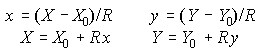where R is the radius of the projection and (X_0,Y_0) the position of the center of the map. With a distance of 70 mm between the marks on the map, R = 160.43 mm. For maps 1 to 3 and 7 to 12, X_0 = 175 mm and Y_0 = 140 mm; for maps 4 to 6, X_0 = 140 mm and Y_0 = 175 mm.

We further define the direction vector of an object by means of the relationships: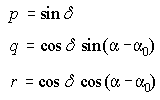where alpha and delta are the right ascension and declination of the object and alpha_0 is the right ascension of the center of the map (given in degrees in Table 1).

Table 1. Right ascension alpha_0, declination delta_0 and cartesian coordinates X_0 and Y_0 of the center of each map in the Gnomonic Atlas Brno 2000.0.

```-------------------------------------------------------------------------------------------------------------------------------
Map        1         2         3         4         5         6         7         8         9        10        11        12
alpha_0   30°      150°      270°       90°      210°      330°       30°      150°      270°       90°      210°      330°
delta_0   55.68°    55.68°    55.68°      4.89°    4.89°     4.89°    -4.89°    -4.89°    -4.89°   -59.29°   -59.29°   -59.29°
X_0      175       175       175       140      140       140        175       175       175       175       175       175
Y_0      140       140       140       175      175       175        140       140       140       140       140       140
-------------------------------------------------------------------------------------------------------------------------------
```

The calculation of the position of an object on the map starts with the calculation of the direction vector using system (2). Then calculate s = p sin delta_0 + r cos delta_0, with delta_0 the declination of the center of the map; the values of delta_0 are also given in Table 1. If s < 0.582, the object cannot be drawn on the map. The standard coordinates of the object are then given by the relationship: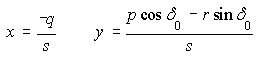from which it is easy to calculate the coordinates (X,Y), using relation (1).

In the other direction, one has to calculate first the standard coordinates using (1) and the polar radius t = sqrt(1+x²+y²). The components of the direction vector are then given by: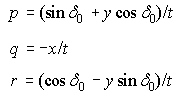from which the values of alpha and delta can be determined using the system in (2)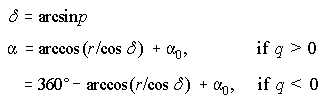Subtract 360° if this value is exceeded by alpha.

### Hints for measuring X, Y coordinates from Atlas Brno

For reporting positional data it is important that the scale of the charts remains unchanged. This means the distance between the thin crosses has to be 70 mm. The origin of the X, Y coordinates is located in the bottom left corner of the chart (X-axis to the right, Y-axis upwards). The reference lines are the inner ones, not the thick lines. Photocopiers often tend to change the original scale. Therefore, use the originals you bought whenever you are going to make copies. Otherwise you merely add to the errors. If the scale error exceeds 3 mm over the whole length of the chart (i.e. if the distance between the thin reference lines differs by more than 3 mm from 280 mm for the short side and/or 350 mm for the long one) you should use another device.

In order to minimize the influence of the remaining scale errors, the coordinates for a given meteor must refer to the same coordinate lines. A ruler of at least 35 cm length is recommended.